# 4.5: Law of Cosines

•• Contributed by CK12
• CK12

The Law of Cosines is a generalized Pythagorean Theorem that allows you to solve for the missing sides and angles of a triangle even if it is not a right triangle. Suppose you have a triangle with sides 11, 12 and 13. What is the measure of the angle opposite the 11?

### The Law of Cosines

The Law of Cosines is:

$$c^{2}=a^{2}+b^{2}-2 a b \cdot \cos C$$

It is important to understand the proof: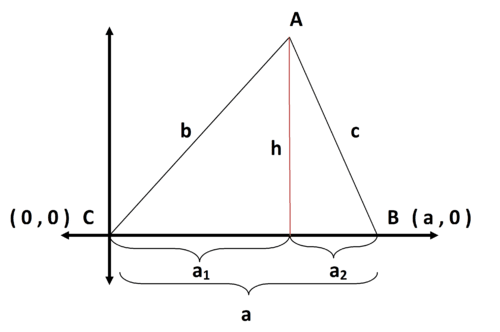You know four facts from the picture:

$a=a_{1}+a_{2}$

$b^{2}=a_{1}^{2}+h^{2}$

$c^{2}=a_{2}^{2}+h^{2}$

$\cos C=\frac{a_{1}}{b}$

Once you verify for yourself that you agree with each of these facts, check algebraically that these next two facts must be true.

$a_{2}=a-a_{1}$

$a_{1}=b \cdot \cos C$

Now the Law of Cosines is ready to be proved using substitution, FOIL, more substitution and rewriting to get the order of terms right.

$$c^{2}=a_{2}^{2}+h^{2} \quad(3$$ again $$)$$
$$c^{2}=\left(a-a_{1}\right)^{2}+h^{2} \quad($$ substitute using 5$$)$$
$$c^{2}=a^{2}-2 a \cdot a_{1}+a_{1}^{2}+h^{2} \quad(\mathrm{FOIL})$$
$$c^{2}=a^{2}-2 a \cdot b \cdot \cos C+a_{1}^{2}+h^{2} \quad($$ substitute using 6$$)$$
$$c^{2}=a^{2}-2 a \cdot b \cdot \cos C+b^{2} \quad($$ substitute using 2$$)$$
$$c^{2}=a^{2}+b^{2}-2 a b \cdot \cos C \quad$$ (rearrange terms)

There are only two types of problems in which it is appropriate to use the Law of Cosines. The first is when you are given all three sides of a triangle and asked to find an unknown angle. This is called SSS (side-side-side) in geometry. The second situation where you will use the Law of Cosines is when you are given two sides and the included angle and you need to find the third side. This is called SAS (side-angle-side).

Take the following triangle.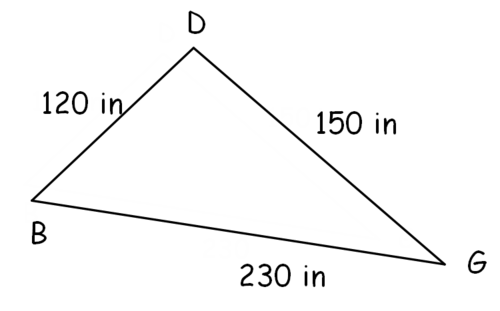The measure of angle $$D$$ is missing and can be found using the Law of cosines. It is necessary to set up the Law of cosines equation very carefully with $$D$$ corresponding to the opposite side of $$230 .$$ The letters are not $$A B C$$ like in the proof, but those letters can always be changed to match the problem as long as the angle in the cosine corresponds to the side used in the left side of the equation.

\begin{aligned} c^{2} &=a^{2}+b^{2}-2 a b \cdot \cos C \\ 230^{2} &=120^{2}+150^{2}-2 \cdot 120 \cdot 150 \cdot \cos D \\ 230^{2}-120^{2}-150^{2} &=-2 \cdot 120 \cdot 150 \cdot \cos D \\ \frac{230^{2}-120^{2}-150^{2}}{-2 \cdot 120 \cdot 150} &=\cos D \\ D &=\cos ^{-1}\left(\frac{230^{2}-120^{2}-150^{2}}{-2 \cdot 120 \cdot 150}\right) \approx 116.4^{\circ} \approx 2.03 \text { radians } \end{aligned}

### Examples

Example 1

Earlier, you were given a triangle with sides 11, 12, and 13 and asked what the measure of the angle opposite 11 is. A triangle that has sides 11, 12 and 13 is not going to be a right triangle. In order to solve for the missing angle you need to use the Law of Cosines because this is a SSS situation.

\begin{aligned} c^{2} &=a^{2}+b^{2}-2 a b \cdot \cos C \\ 11^{2} &=12^{2}+(13)^{2}-2 \cdot 12 \cdot 13 \cdot \cos C \\ C &=\cos ^{-1}\left(\frac{11^{2}-12^{2}-13^{2}}{-2 \cdot 12 \cdot 13}\right) \approx 52.02^{\circ} \end{aligned}

Example 2

Determine the length of side $$p$$.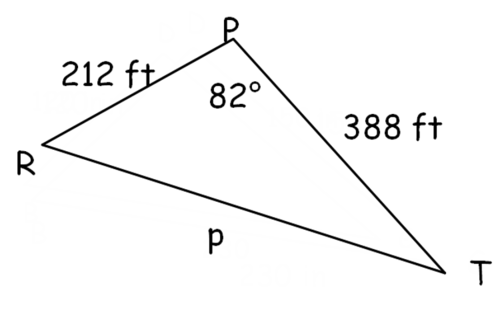\begin{aligned} c^{2} &=a^{2}+b^{2}-2 a b \cdot \cos C \\ p^{2} &=212^{2}+388^{2}-2 \cdot 212 \cdot 388 \cdot \cos 82^{\circ} \\ p^{2} & \approx 172592.354815 \\ p & \approx 415.44 \end{aligned}

Example 3

Determine the degree measure of angle $$N$$.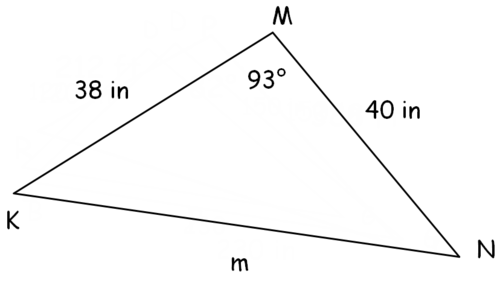This problem must be done in two parts. First apply the Law of cosines to determine the length of side $$m$$. This is a SAS situation like Example B. Once you have all three sides you will be in the SSS situation like in Example $$A$$ and can apply the Law of cosines again to find the unknown angle $$N$$.

\begin{aligned} c^{2} &=a^{2}+b^{2}-2 a b \cdot \cos C \\ m^{2} &=38^{2}+40^{2}-2 \cdot 38 \cdot 40 \cdot \cos 93^{\circ} \\ m^{2} & \approx 3203.1 \\ m & \approx 56.59 \end{aligned}

Now that you have all three sides you can apply the Law of cosines again to find the unknown angle $$N$$. Remember to match angle $$N$$ with the corresponding side length of 38 inches. It is also best to store $$m$$ into your calculator and use the unrounded number in your future calculations.

\begin{aligned} c^{2} &=a^{2}+b^{2}-2 a b \cdot \cos C \\ 38^{2} &=40^{2}+(56.59)^{2}-2 \cdot 40 \cdot(56.59) \cdot \cos N \\ 38^{2}-40^{2}-(56.59)^{2} &=-2 \cdot 40 \cdot(56.59) \cdot \cos N \\ \frac{38^{2}-40^{2}-(56.59)^{2}}{-2 \cdot 40 \cdot(56.59)} &=\cos N \\ N &=\cos ^{-1}\left(\frac{38^{2}-40^{2}-(56.59)^{2}}{-2 \cdot 40 \cdot(56.59)}\right) \approx 42.1^{\circ} \end{aligned}

For the next two examples, use the triangle below.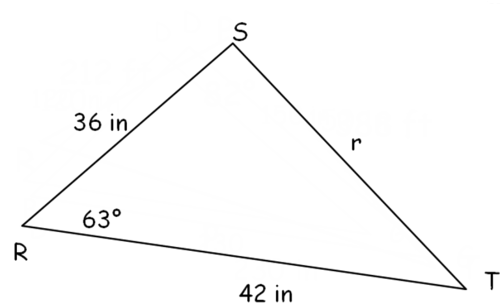Example 4

Determine the length of side $$r$$.

$$r^{2}=36^{2}+42^{2}-2 \cdot 36 \cdot 42 \cdot \cos 63$$

$$r \approx 41.07$$

Example 5

Determine the length of side $$r$$.

$$r^{2}=36^{2}+42^{2}-2 \cdot 36 \cdot 42 \cdot \cos 63$$

$$r \approx 41.07$$

Review

For all problems, find angles in degrees rounded to one decimal place.

In $$\Delta A B C, a=12, b=15,$$ and $$c=20$$

1. Find the measure of angle $$A$$.

2. Find the measure of angle $$B$$.

3. Find the measure of angle $$C$$.

4. Find the measure of angle $$C$$ in a different way.

In $$\Delta D E F, d=20, e=10,$$ and $$f=16$$

5. Find the measure of angle $$D$$.

6. Find the measure of angle $$E$$.

7. Find the measure of angle $$F$$.

In $$\Delta G H I, g=19, \angle H=55^{\circ},$$ and $$i=12$$

8. Find the length of $$h$$.

9. Find the measure of angle $$G$$.

10. Find the measure of angle $$I$$.

11. Explain why the Law of cosines is connected to the Pythagorean Theorem.

12. What are the two types of problems where you might use the Law of cosines?

Use the Law of cosines to determine whether or not each triangle is possible.

13. $$a=5, b=6, c=15$$

14. $$a=1, b=5, c=4$$

15. $$a=5, b=6, c=10$$

...